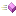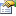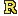The following tables list the members exposed by TAgk.

 Name DescriptionReturns absolute of a value, that is the positive version of the number.Returns the arccosine of a value in degrees.Returns the arccosine of a value in radians.Returns the arcsine of a value in degrees.Returns the arcsine of a value in radians.Returns the arctangent of a value in degrees.This is the overview for the ATanFull method overload.This is the overview for the ATanFullRad method overload.Returns the arctangent of a value in radians.Rounds a float to the next highest integer. This differs from Trunc when using positive numbers: Trunc(1.6) is 1 but Ceil(1.6) is 2.Returns the cosine of a value in degrees.Returns the cosine of a value in radians.Outputs an error message.Rounds a float to the next lowest integer. This differs from Trunc when using negative numbers: Trunc(-1.6) is -1 but Floor(-1.6) is -2.Returns True if an error occured since the last call to this method. False if not.Returns a string describing the last AGK error that occurred.This is the overview for the Modulus method overload.This is the overview for the Random method overload.Will randomly negate the value given and return it.Rounds a float to the nearest integer (positive or negative).Sets the error mode for the AGK from ignore, report, or stop from AGK commands.Sets the seed for the random number generator. Two AGK applications using the same seed value will generate the same sequence of random numbers.Returns the sine of a value in degrees.Returns the sine of a value in radians.Returns the square root of a float value.Returns the tangent of a value in degrees.Returns the tangent of a value in radians.Converts a TAgkDeviceOrientation enumeration to a string value.Returns the integer part of a float value with no rounding either way.Outputs a warning message.
 Name DescriptionThe height in pixels of the current device's screen.A string containing the name of the current platform. e.g. 'windows' or 'bada'.The current orientation of the device.The width in pixels of the current device's screen.Returns the current error mode.